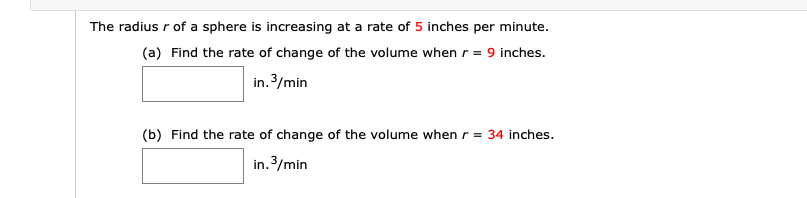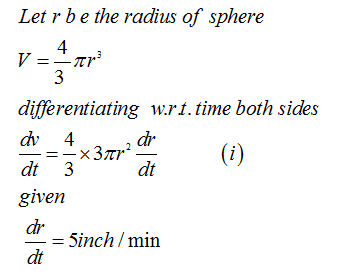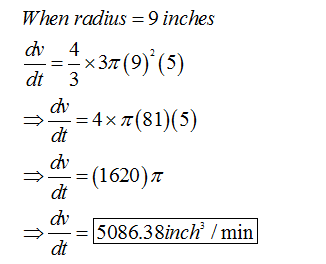# The radius r of a sphere is increasing at a rate of 5 inches per minute.(a) Find the rate of change of the volume when r = 9 inches.in. 3/min(b) Find the rate of change of the volume whenr = 34 inches.in.3/min

Question
22 viewshelp_outlineImage TranscriptioncloseThe radius r of a sphere is increasing at a rate of 5 inches per minute. (a) Find the rate of change of the volume when r = 9 inches. in. 3/min (b) Find the rate of change of the volume when r = 34 inches. in.3/min fullscreen
check_circle

Step 1

The radius r of a sphere is increasing at a rte of 5 onches pe rminute

1. Find the rate of change of the volume when r= 9 inches
2. Fin the rate of change of the volume when r =34 inches
Step 2Step 3...

### Want to see the full answer?

See Solution

#### Want to see this answer and more?

Solutions are written by subject experts who are available 24/7. Questions are typically answered within 1 hour.*

See Solution
*Response times may vary by subject and question.
Tagged in